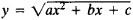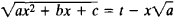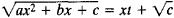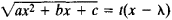Euler Substitutions

The following article is from The Great Soviet Encyclopedia (1979). It might be outdated or ideologically biased.

Euler Substitutions

substitutions used to reduce integrals of the form

R(x, y) dx

to integrals of rational functions, whereand R(x, y) is a rational function of x and y. The substitutions were proposed by L. Euler in 1768.

The first Euler substitution isand is applicable if a > 0. The second Euler substitution isand is applicable if c > 0. The third Euler substitution iswhere λ is one of the roots of the trinomial ax2 + bx + c; this substitution is applicable if the roots of the trinomial are real. In practice, Euler substitutions require cumbersome transformations, and various artificial procedures that simplify calculation are normally used instead of them.

Similar substitutions are made in number theory in the solution of indeterminate equations of second degree in rational numbers.